• 【强化学习】值迭代和策略迭代 在强化学习中我们经常会遇到策略迭代与值迭代，但是很多人都搞不清楚他们两个之间的区别，他们其实都是强化学习中的动态规划方法（DP）。 ——《Reinforcement Learning:An ...

【强化学习】值迭代和策略迭代

在强化学习中我们经常会遇到策略迭代与值迭代，但是很多人都搞不清楚他们两个之间的区别，他们其实都是强化学习中的动态规划方法（DP）。 ——《Reinforcement Learning:An Introduction》

（一）值迭代
对每一个当前状态 s ,对每个可能的动作 a 都计算一下采取这个动作后到达的下一个状态的期望价值。看看哪个动作可以到达的状态的期望价值函数最大，就将这个最大的期望价值函数作为当前状态的价值函数 V(s) ，循环执行这个步骤，直到价值函数收敛。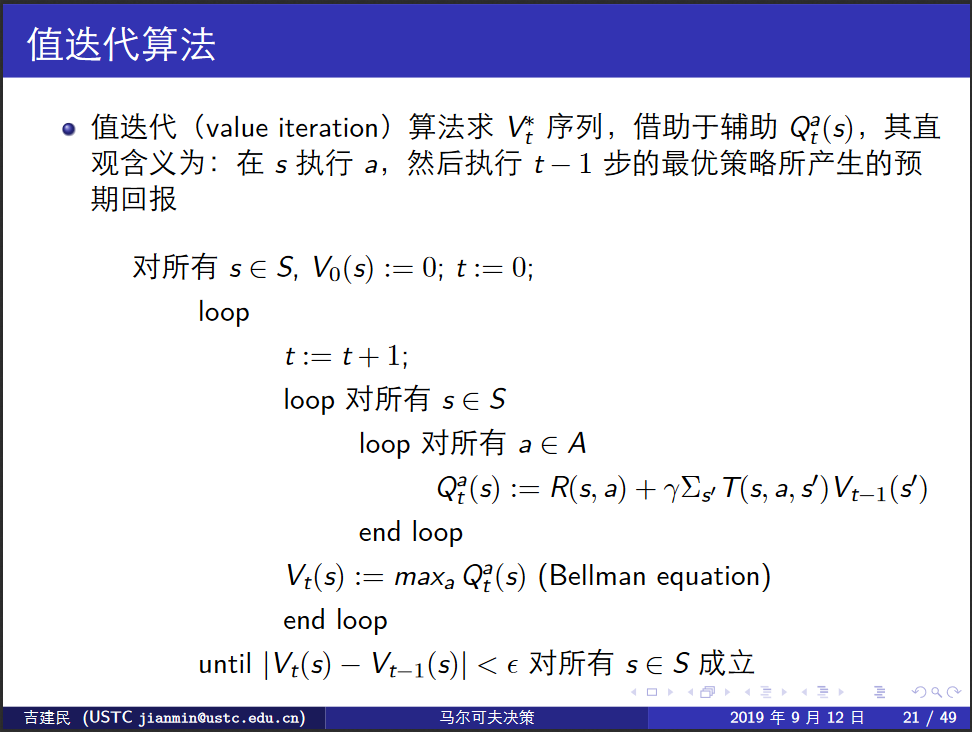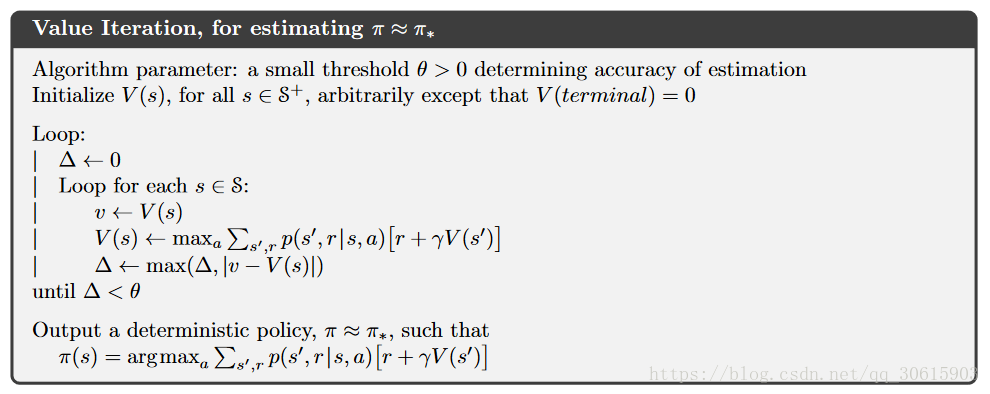（二）策略迭代

从一个初始化的策略出发，先进行策略评估，然后改进策略，评估改进的策略，再进一步改进策略，经过不断迭代更新，直达策略收敛，这种算法被称为“策略迭代”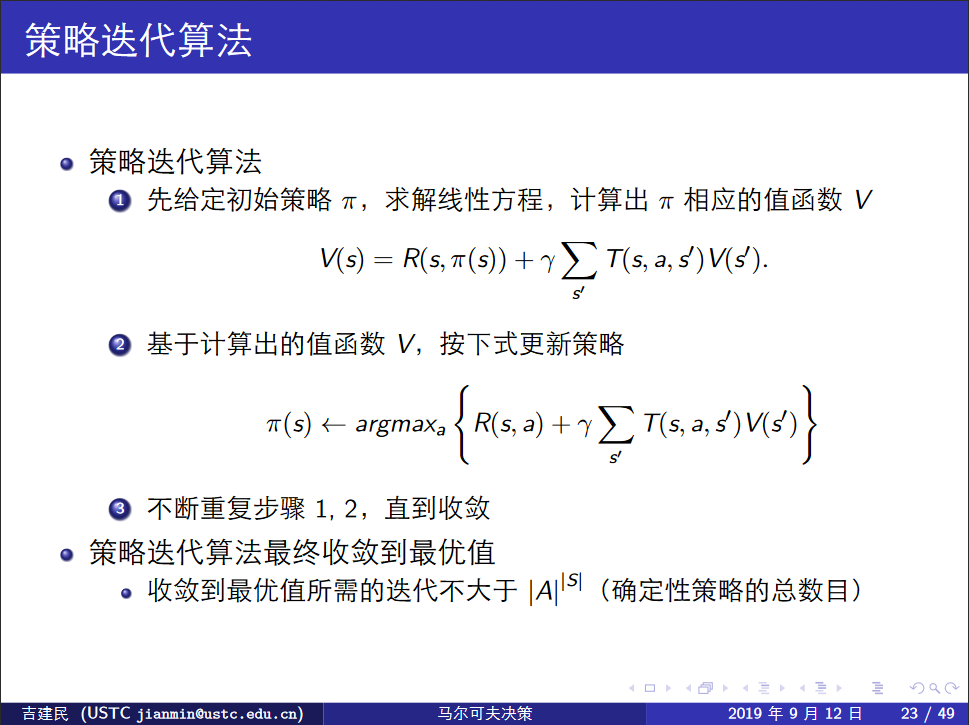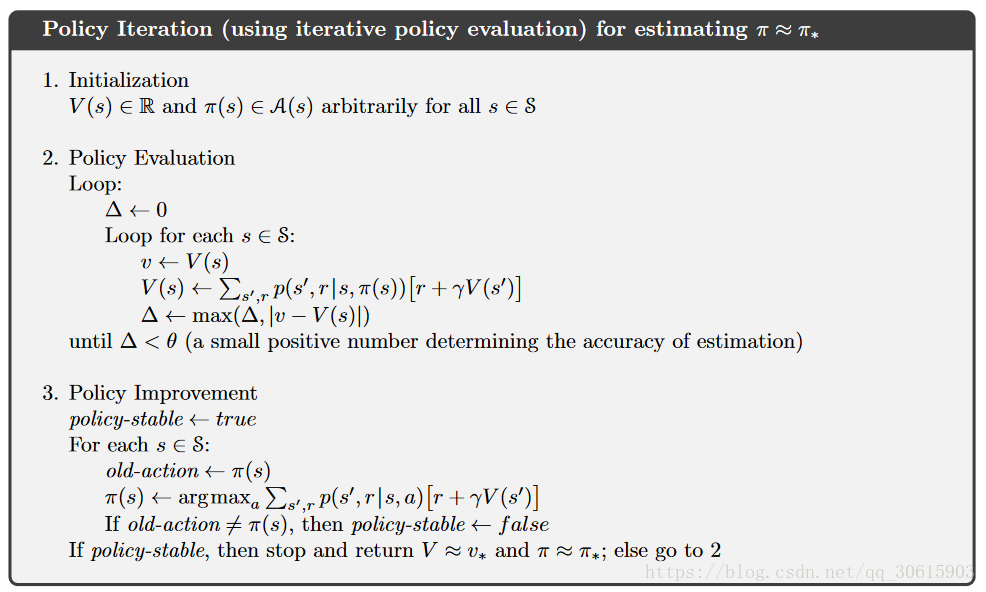References:
 【强化学习】值迭代与策略迭代

转载于:https://www.cnblogs.com/xxxxxxxxx/p/11536460.html
展开全文• 在强化学习中我们经常会遇到策略迭代与值迭代，但是很多人都搞不清楚他们两个之间的区别，他们其实都是强化学习中的动态规划方法。 科普：动态规划dynamic programming简称（DP） 【强化学习】值迭代与策略...

引自Reinforcement Learning:An Introduction强化学习名著2018新编版
在强化学习中我们经常会遇到策略迭代与值迭代，但是很多人都搞不清楚他们两个之间的区别，他们其实都是强化学习中的动态规划方法。
科普：动态规划dynamic programming简称（DP）

【强化学习】值迭代与策略迭代
在强化学习中，要求一个完全已知的环境模型，所谓完全已知，就是MDP的五元组全部已知，当然了，主要还是指状态转移概率已知。这种学习方式就是有模型学习(Model-based learning)，由于他们假设了一个完全已知的模型并且他们的计算代价很高，所以在强化学习中他们的作用是有限，但DP仍然为理解值迭代和策略迭代两个方法提供了一个必要的基础。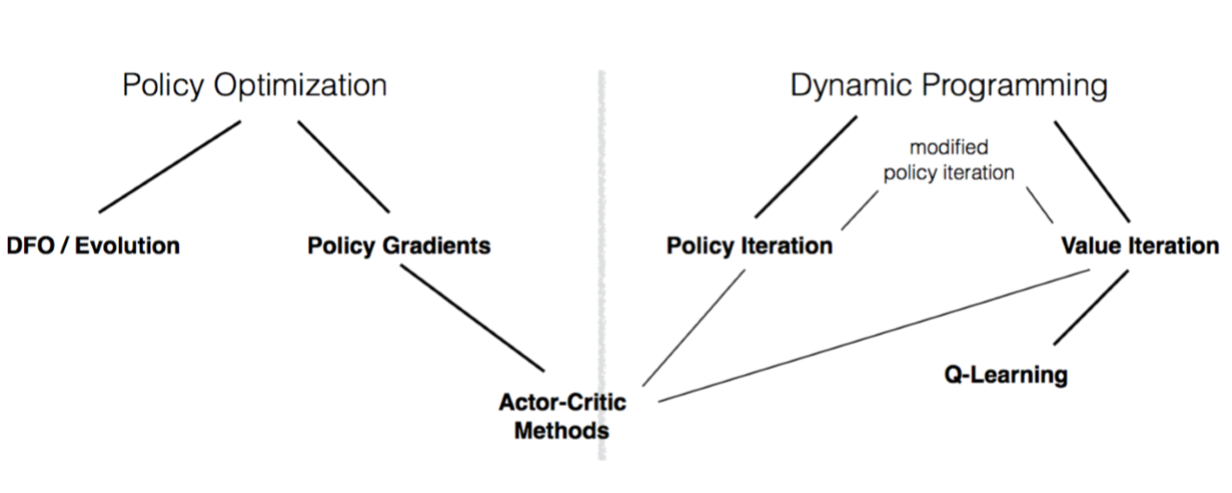1、策略迭代 POLICY ITERATION
从一个初始化的策略出发，先进行策略评估，然后改进策略，评估改进的策略，再进一步改进策略，经过不断迭代更新，直达策略收敛，这种算法被称为“策略迭代”
1.1、 策略预估Policy Evaluation (Prediction)
策略评估就是计算任意策略的状态值函数$V_\pi$，也就是对当前策略下计算出每个状态下的状态值，这就是策略预估，我们也把它称为预测问题。他的计算方式参考之前我们提到过得状态值函数
$V_\pi(s) = E_\pi[G_t|S_t=s]$
$V_\pi(s) = E_\pi[R_{t+1}+\gamma G_{t+1}|S_t=s]$
$V_\pi(s) = E_\pi[R_{t+1}+\gamma V(S_{t+1})|S_t=s]$
$V_\pi(s) = \sum_a\pi(a|s)\sum_{s'}P(s'|s,a)(R(s,a,s′)+γV_\pi(s′))$
因为是策略迭代所以此处的$\pi(a|s)$即为在 s 状态下选取  a 动作的可能，所以策略迭代的公式应该为
$V_{k+1}(s) = \sum_a\pi(a|s)\sum_{s',r}P(s',r|s,a)(r+γV_k(s′))$
举例说明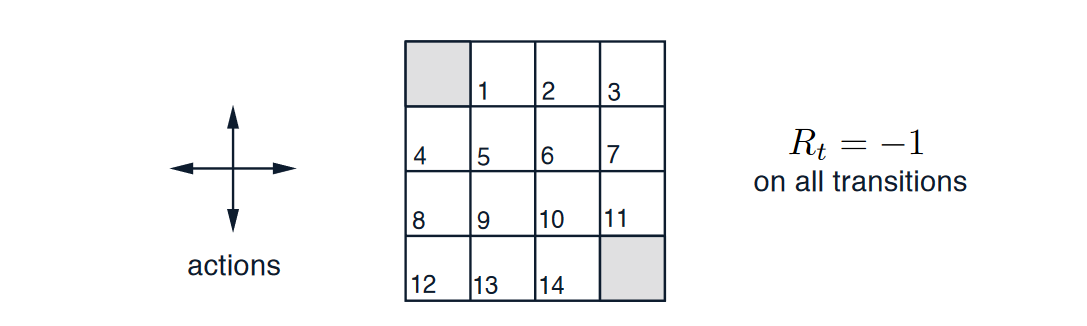如图所示，现在存在4*4的区域每个格子代表一个状态编号为{1,2…14},动作空间为{上，下，左，右}，将Rt都初始化为-1。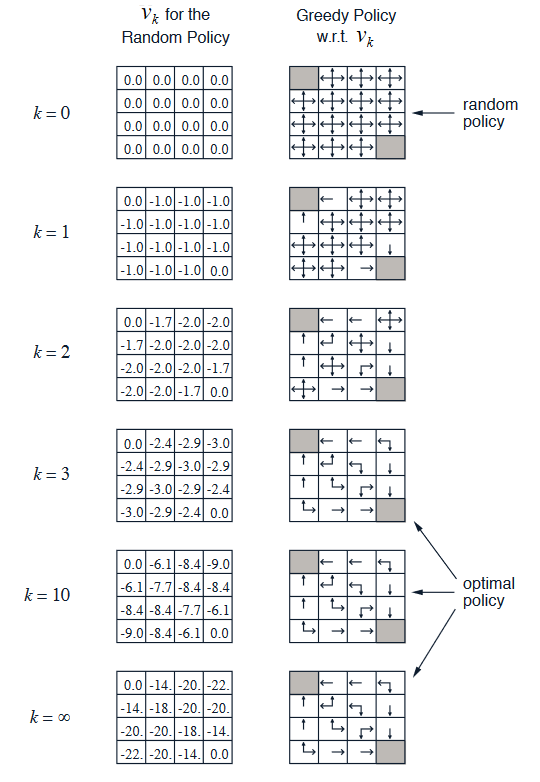通过上图我们可以很清晰的看出完整的估算过程，一开始的随机策略每个状态 s 都是有{上,下,左,右}四个动作，然后在经过每轮估算的时候是根据每个状态 s 分别对当前状态下可选取的动作获得的回报进行预估，然后在其中选择出获得回报最大的动作保留 也就是增加动作被选择的概率。
1.2、 策略改进Policy Improvement
我们计算策略的价值函数的原因是了帮助找到更好的策略。因为我们之前进行了策略评估得到了上一个策略下的每个状态下的状态值，所以接下来就要根据这些状态值对策略进行改进，计算出新的策略。计算方式如下
在每个状态s时，对每个可能的动作a,都计算一下采取这个动作后到达的下一个状态的期望价值。看看哪个动作可以到达的状态的期望价值函数最大，就选取这个动作。以此更新了π(s)
计算期望公式
$Q_\pi(s,a) = \sum_{s',r}P(s',r|s,a)(r+\gamma V_\pi(s'))$
改进策略
$\pi'(s) = argmax_aQ_\pi(s,a)$
改进策略后更新状态值
$V_{\pi'}(s) = max_a\sum_{s',r}P(s',r|s,a)(r+\gamma V_{\pi'}(s'))$
所以经过以上两个步骤就组成了策略迭代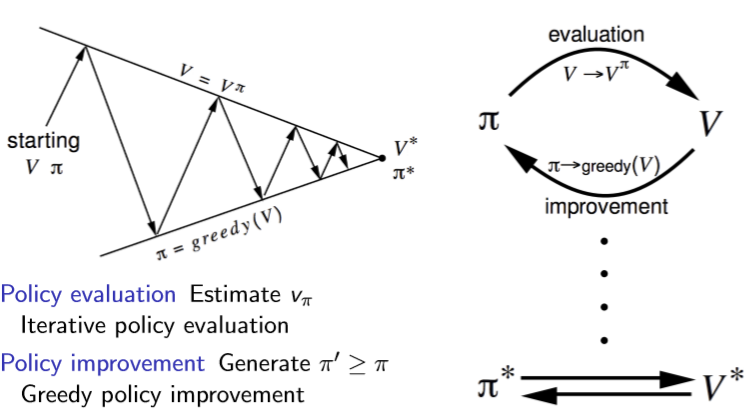算法伪代码如下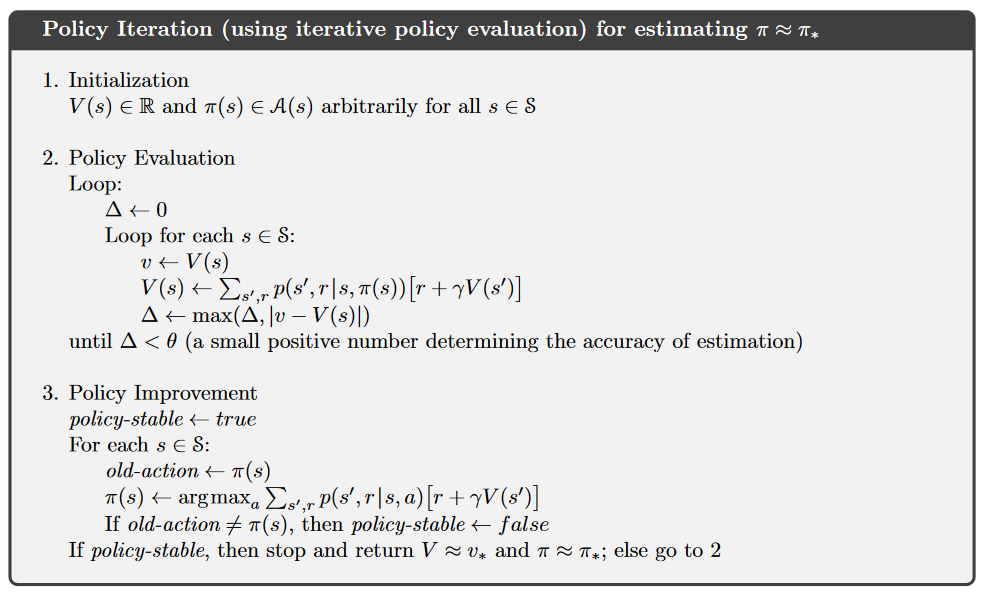2、价值迭代 VALUE ITERATION
这个就没什么过于复杂的东西，和上面差不多，对每一个当前状态 s ,对每个可能的动作 a 都计算一下采取这个动作后到达的下一个状态的期望价值。看看哪个动作可以到达的状态的期望价值函数最大，就将这个最大的期望价值函数作为当前状态的价值函数 $V(s)$ 循环执行这个步骤，直到价值函数收敛。
期望值计算公式
$V_{k+1}(s) = max_a\sum_{s',r}P(s',r|s,a)(r+\gamma V_{k}(s'))$
也就是说与策略迭代不同的是值迭代是根据状态期望值选择动作，而策略迭代是先估计状态值然后修改策略。
伪代码如下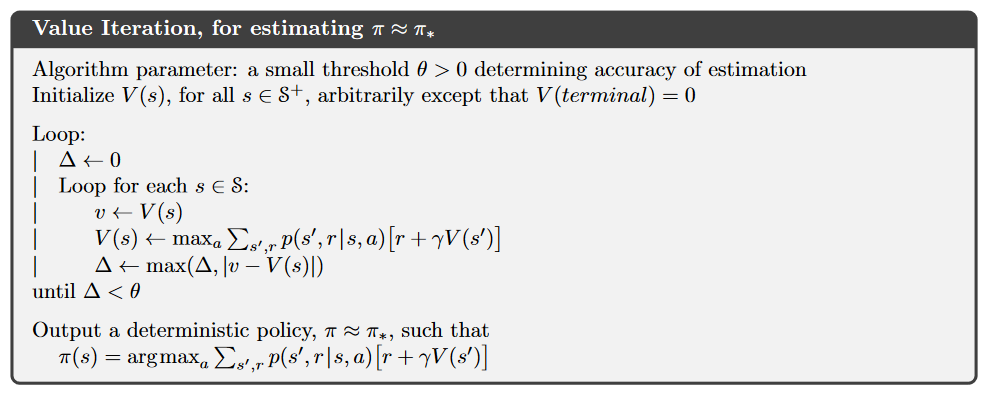展开全文• ## 策略迭代与值迭代的区别

万次阅读 多人点赞 2017-08-31 20:35:46
策略迭代与值迭代都属于强化学习里面策略求解中的动态规划方法。其区别是什么呢？ 首先看一张图片： 首先看策略迭代： １.initialization 初始化所有状态的v(s)以及π(s)（初始化为随机策略） 2....
策略迭代与值迭代都属于强化学习里面策略求解中的动态规划方法。其区别是什么呢？

首先看一张图片：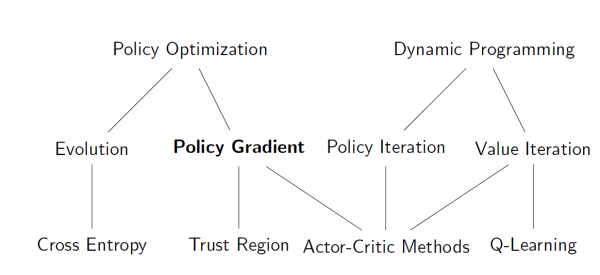首先看策略迭代：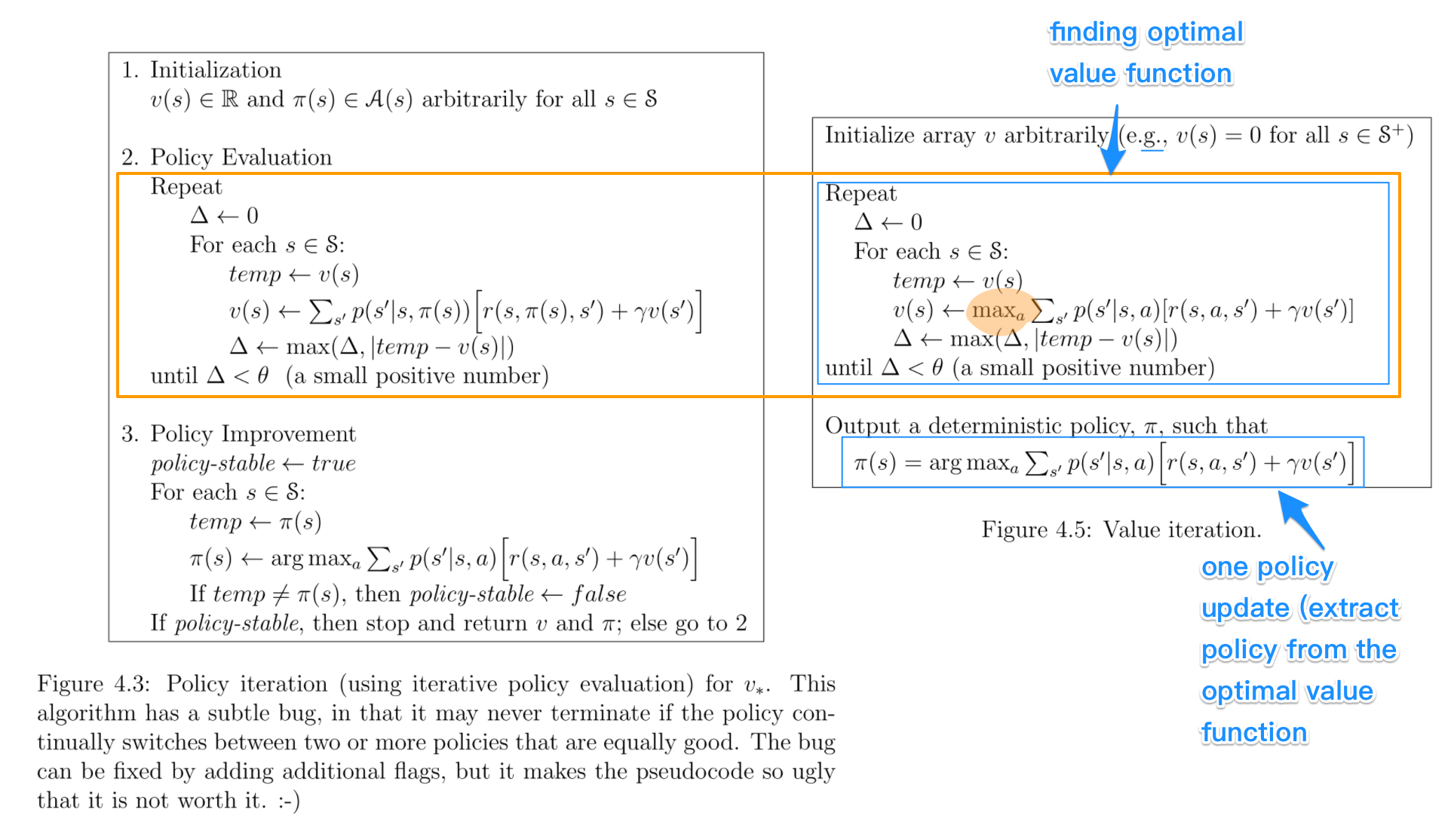１.initialization
初始化所有状态的v(s)以及π(s)（初始化为随机策略） 2.poicy evaluation
用当前的v(s)对当前策略进行评估，计算出每一个状态的v(s)，直到v(s)收敛，才算训练好了这个状态价值函数V(s) 3.policy improvement
既然上一步已经得到了当前策略的评估函数V(s),那么就可以利用这个评估函数进行策略改进啦。
在每个状态s时，对每个可能的动作a,都计算一下采取这个动作后到达的下一个状态的期望价值。看看哪个动作可以到达的状态的期望价值函数最大，就选取这个动作。以此更新了π(s)
然后再次循环上述２、３步骤，直到V(s)与π(s)都收敛。
正如下面所说:

In Policy Iteration algorithms, you start with a random policy, then find the value function of that policy (policy evaluation step), then find an new (improved) policy based on the previous value function, and so on. In this process, each policy is guaranteed to be a strict improvement over the previous one (unless it is already optimal). Given a policy, its value function can be obtained using the Bellman operator.

再来看值迭代： １.initialization
初始化所有状态的v(s) ２.finding optimal value function（找到最优的价值函数）
注意伪代码里的max，对每一个当前状态s,对每个可能的动作a,都计算一下采取这个动作后到达的下一个状态的期望价值。看看哪个动作可以到达的状态的期望价值函数最大，就将这个最大的期望价值函数作为当前状态的价值函数v(s)    循环执行这个步骤，直到价值函数收敛,就可以得到最优optimal的价值函数了 3.policy extraction
利用上面步骤得到的optimal价值函数和状态转移概率，就计算出每个状态应该采取的optimal动作,这个是deterministic呦。

In Value Iteration, you start with a randon value function and then find a new (improved) value function in a iterative process, until reaching the optimal value function. Notice that you can derive easily the optimal policy from the optimal value function. This process is based on the Optimality Bellman operator.

区别及联系

1.策略迭代的第二步policy evaluation与值迭代的第二步finding optimal value function十分相似，除了后者用了max操作，前者没有max.因此后者可以得出optimal value function, 而前者不能得到optimal function.
2.策略迭代的收敛速度更快一些，在状态空间较小时，最好选用策略迭代方法。当状态空间较大时，值迭代的计算量更小一些。
展开全文强化学习
• 上一次已经分享了强化学习的概念以及基本的MDP，本节将分享基于Bellman方程和动态规划的策略迭代和值迭代，对于Bellman方程，大家都比较清楚了，那么我们先介绍一下动态规划算法的基本原理 一、动态规划 这里面我...
本文参考http://www0.cs.ucl.ac.uk/staff/D.Silver/web/Teaching.html

上一次已经分享了强化学习的概念以及基本的MDP，本节将分享基于Bellman方程和动态规划的策略迭代和值迭代，对于Bellman方程，大家都比较清楚了，那么我们先介绍一下动态规划算法的基本原理

一、动态规划

这里面我要简单介绍一下动态规划，因为严格来说，值迭代与策略迭代是用来解决动态规划问题的两种规划方法。而强化学习又有另外一个昵称——就是拟动态规划。说白了强化学习就是模拟动态规划算法。

用一句话来总结动态规划就是，对一个复杂问题给出一个一般性的解决办法。它主要由两个性质:

1、最优子结构：最优解法能被分解到多个子问题中

2、重叠子问题：子问题能重复多次且解法能被重复利用

马尔科夫决策过程（MDP）满足以上两个性质，所以任何MDP都可以用动态规划来解。动态规划与强化学习的区别就是动态规划假设MDP模型是全知的（即参数可知） 而强化学习可以使MDP未知。

MDP需要解决的问题有两种，第一种是prediction，它已知MDP的S,A,P,R,γ以及policy，目标是算出在每个状态下的value function(值函数其实就是问题的目标，一般都是跟reward有关的函数，例如Atari小游戏，一般值函数就是累计的得分的期望。目标一般就是最大化这个值函数。而第二种是control，它已知MDP的S,A,P,R,γ但是policy未知（即动作action未知），因此它的目标不仅是计算出最优的value function而且要给出最优的Policy。

二、策略迭代POLICY ITERATION

策略迭代就是在policy未知的情况下，根据每次的reward学到最优policy。策略迭代每次先初始化每个状态的值函数v(s)和一个策略，然后根据这个策略计算值函数v(s), 通过这个值函数来根据贪心策略更新策略，不断迭代直到策略收敛，下面是算法的流程：

1、initialization

初始化所有状态的v(s)以及π(s)（初始化为随机策略）

2、poicy evaluation

用当前的v(s)对当前策略进行评估，计算出每一个状态的v(s)，直到v(s)收敛，才算训练好了这个状态价值函数V(s)

3、policy improvement

既然上一步已经得到了当前策略的评估函数V(s),那么就可以利用这个评估函数进行策略改进啦。

在每个状态s时，对每个可能的动作a,都计算一下采取这个动作后到达的下一个状态的期望价值。看看哪个动作可以到达的状态的期望价值函数最大，就选取这个动作。以此更新了π(s)

然后再次循环上述２、３步骤，直到V(s)与π(s)都收敛。

下面总结一下Policy evaluation和Policy improvement这两个阶段：

2.1策略评估Policy evaluation

策略评估就是在任意策略下，计算每个状态的值函数vπ，我们也把它称为预测问题。由值函数的定义Vπ​(s)=Eπ​[Gt​∣St​=s]展开为

Vπ​(s)=∑a​π(a∣s)∑s′​P(s′∣s,a)(R(s,a,s′)+γVπ​(s′))

其中π(a∣s)为在s状态下执行动作a的概率，

下面是策略评估的python代码

def policy_evaluation(self, agent, max_iter=-1):

iteration = 0

# iterative eval

while True:

# one iteration

iteration += 1

new_value_pi = agent.value_pi.copy()

for i in range(1, agent.s_len):  # for each state

value_sas = []

ac = agent.pi[i]

transition = agent.p[ac, i, :]

value_sa = np.dot(transition, agent.r + agent.gamma * agent.value_pi)

new_value_pi[i] = value_sa  # value_sas[agent.policy[i]]

diff = np.sqrt(np.sum(np.power(agent.value_pi - new_value_pi, 2)))

# print 'diff={}'.format(diff)

if diff < 1e-6:

break

else:

agent.value_pi = new_value_pi

if iteration == max_iter:

break

2.2策略改进Policy improvement

策略改进是Greedy policy，它寻找每个状态下得到最大值函数vπ的策略π，也就是说，采取哪个动作可以达到下一个状态的最大值，那么就选择这个动作为最优策略

计算期望值

Qπ​(s,a)=∑s′,r​P(s′,r∣s,a)(r+γVπ​(s′))

改进策略

π′(s)=argmaxa​Qπ​(s,a)

改进策略后更新状态值

Vπ′​(s)=maxa​∑s′,r​P(s′,r∣s,a)(r+γVπ′​(s′))

策略改进的python代码如下：

def policy_improvement(self, agent):

new_policy = np.zeros_like(agent.pi)

for i in range(1, agent.s_len):

for j in range(0, agent.a_len):

agent.value_q[i, j] = np.dot(agent.p[j, i, :], agent.r + agent.gamma * agent.value_pi)

max_act = np.argmax(agent.value_q[i, :])

new_policy[i] = max_act

if np.all(np.equal(new_policy, agent.pi)):

return False

else:

agent.pi = new_policy

return True

策略迭代算法的伪代码如下所示：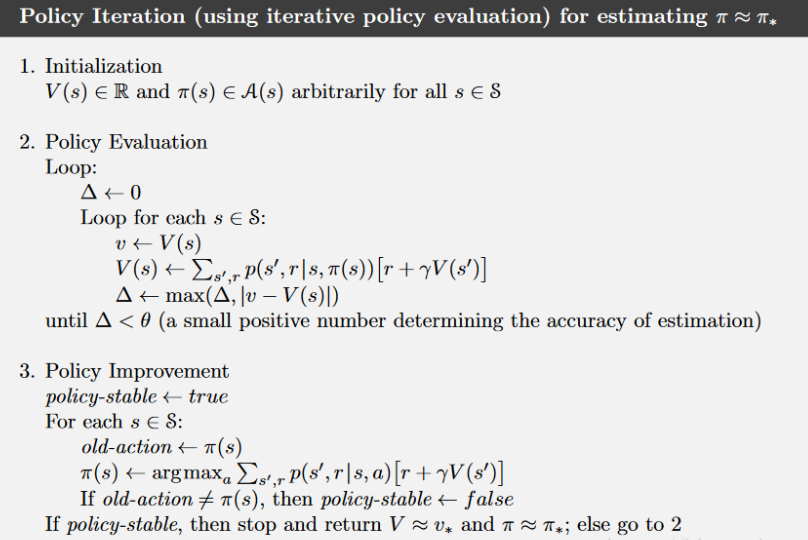三、价值迭代VALUE ITERATION

价值迭代算法主要流程如下。

1、初始化Value Function，即对每个状态的价值都赋一个初始值，一般是0

2、计算每一个状态-动作pair 的值，也就是Q(s,a)

3、 每一个状态st​下都有一个动作空间at​∈A，maxat​​Q(st​,at​)，即最大的Q值作为当前状态的价值，更新Value Function

4、返回第2步，直至Value Function收敛。

迭代公式如下：

Vt+1​(s)=max​∑s′,r​P(s′,r∣s,a)(r+γVt​(s′))

值迭代的伪代码如下：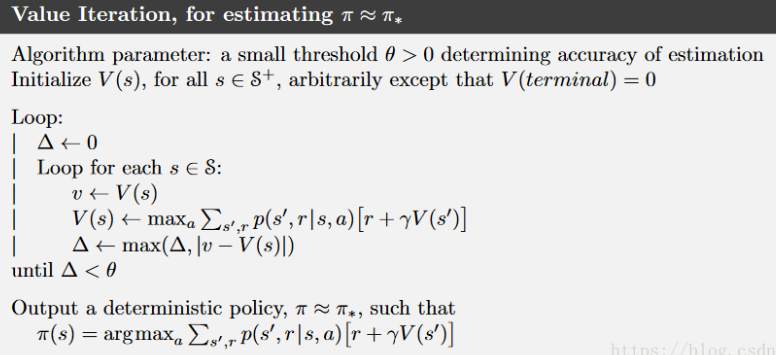值迭代的python代码如下：

def value_iteration(self, agent, max_iter=-1):

iteration = 0

while True:

iteration += 1

new_value_pi = np.zeros_like(agent.value_pi)

for i in range(1, agent.s_len):  # for each state

value_sas = []

for j in range(0, agent.a_len):  # for each act

value_sa = np.dot(agent.p[j, i, :], agent.r + agent.gamma * agent.value_pi)

value_sas.append(value_sa)

new_value_pi[i] = max(value_sas)

diff = np.sqrt(np.sum(np.power(agent.value_pi - new_value_pi, 2)))

if diff < 1e-6:

break

else:

agent.value_pi = new_value_pi

if iteration == max_iter:

break

print('Iter {} rounds converge'.format(iteration))

for i in range(1, agent.s_len):

for j in range(0, agent.a_len):

agent.value_q[i, j] = np.dot(agent.p[j, i, :], agent.r + agent.gamma * agent.value_pi)

max_act = np.argmax(agent.value_q[i, :])

agent.pi[i] = max_act

那么策略迭代和值迭代有什么区别和联系呢？

共同点：

他们都是基于模型的方法，也就是说都需要知道环境的状态转移概率P；
都需要通过bellman方程来更新状态值函数
不同点：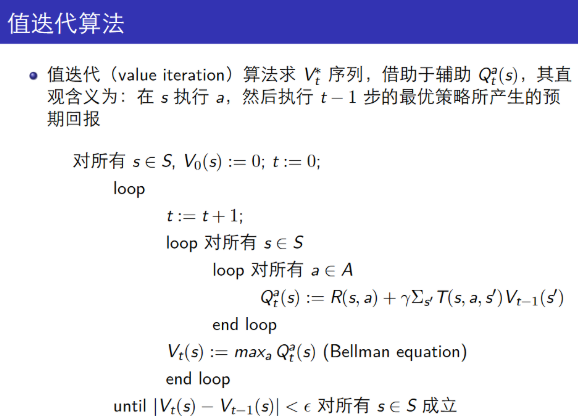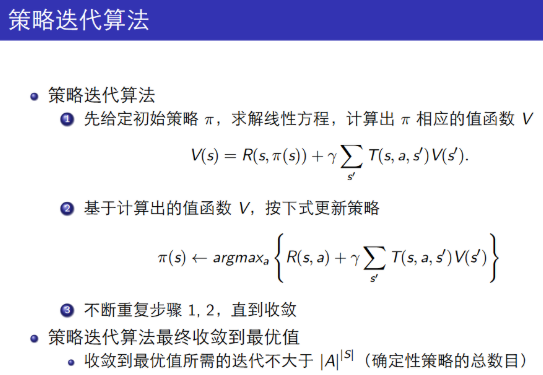1、策略迭代在价值评估阶段，每迭代一次都需要保证每个状态的值函数收敛，这是非常耗时的；而值迭代是采用动态规划的思想来收敛每个状态的值函数的。

2、策略迭代的第二步policy evaluation与值迭代的第二步finding optimal value function十分相似，除了后者用了max操作，前者没有max.因此后者可以得出optimal value function, 而前者不能得到optimal function.

3、策略迭代的收敛速度更快一些，在状态空间较小时，最好选用策略迭代方法。当状态空间较大时，值迭代的计算量更小一些。

4*****、侧重点不同：策略迭代最后是策略收敛，而值迭代是值函数收敛；收敛的方式也不同，策略迭代是argmax，而值函数是max


展开全文强化学习
• 强化学习实战（一）：用值迭代和策略迭代解决Frozen Lake问题Frozen Lake 问题值迭代(Value Iteration)和策略迭代(Policy Iteration)CodeReference Frozen Lake 问题 Winter is here. You and your friends were ...
• 上一节我们说了马尔可夫决策过程，它是对完全可观测的环境进行描述的，也就是观测到的内容完整决定了决策所...这种方法我们通常称其为值迭代；2. 或者直接寻找最优策略和最优价值函数，这种方法称为策略迭代。动态规划
• 本篇博客对“有模型学习”的两种方法进行介绍，分别是策略迭代和值迭代。 我们之前已经说到了MDP可以表示成一个元组（X, A, Psa, R），我们对最优策略的求解方法自然也就与这个元组密切相关：如果该过程的四元组均为...机器学习 增强学习
• 这次我们来学习求解强化学习模型的2种思路——值迭代与策略迭代动态规划这里面我要简单介绍一下动态规划，因为严格来说，值迭代与策略迭代是用来解决动态规划问题的两种规划方法。而强化学习又有另外一个昵称——...动态规划 强化学习 算法
•   在这个系列的马尔可夫决策过程与动态规划这篇文章中我们是有说这个策略迭代和值迭代的，但是说的更公式化一点，本文主要是对其进行一个更加深刻的理解。 为什么需要值迭代   其实我们更希望强化学习直接学习...
• ## 值迭代、策略迭代

千次阅读 2017-04-29 13:22:35
函数策略: 状态s到动作a的映射：π:S→A\pi:S\rightarrow A 函数：函数都是对应于特定的策略的，即VπV^\pi 对于策略π\pi，状态s的函数：Vπ(s)=E[R(s0)+γR(s1)+γ2R(s2)+...|s0=s,π].V^\pi(s)=E[R(s_0...强化学习 机器学习 人工智能
• 《深入浅出强化学习原理入门》学习笔记（五）基于Python和gym实现策略迭代方法和值迭代方法1、策略迭代方法策略评估策略改善值迭代方法最优控制和强化学习比较 1、策略迭代方法 python代码包括策略评估和策略改善两...python 机器学习 人工智能 强化学习
• 强化学习有两种常见迭代训练算法：策略迭代算法和值迭代算法。在上一篇博客&amp;lt;&amp;lt;强化学习笔记（二）&amp;gt;&amp;gt;中已经详细描述了策略迭代算法，其实值迭代算法和策略迭代算法的基本...
• 在循环一次后，停止策略评估的步骤就是值迭代 伪代码如下 如果帮到你强化学习 python 算法 深度学习
• 优点：迭代器存储的是生成数据的方式（可以迭代的对象），并不是存储结果，很有效的减少内存的使用。 注意：可以迭代的对象不一定是迭代器，但是迭代器一定可以迭代迭代器一定含有iter()和next()方法 判断...
• 强化学习-在线可视化-值迭代-karpathy 文章目录强化学习-在线可视化-值迭代-karpathy前言：网页链接： 前言： 之前因为好奇值迭代的具体过程，所以搜了一下可视化的工具和内容。 发现了这个特别好使的网页！ 虽然我...强化学习 可视化
• 重新回顾一下值迭代算法（原理见3.1 基于模型的动态规划方法）： 进行策略改善之前不一定要等到策略值函数收敛，可以在评估一次后就进行策略改善，迭代的终止条件是值函数不再发生变化（就是每个状态下的值函数不再...强化学习
• 马尔克服决策过程可以用方程组求解简单问题，但是对于复杂一点的问题，一般通过迭代的思想对其进行求解。动态规划是非常有效的求解马尔可夫决策过程的方法。   动态规划大体思想：在已知模型的基础之上判断策略的...
• Bellman找到了一种方法来估计任何状态s的最佳状态（*），记为V*(s)，V*(s)是代理在达到状态s时（假设采取最佳行为）所期望的所有折价未来奖励的总和。 他表明，如果代理能发挥最佳作用，则适用Bellman最优性方程。...
• 近期在学习人工智能课程的时候接触到了强化学习(Reinforcement Learning)，并介绍到了一种叫做MDP(马尔可夫决策)的思想，最终布置了个Grid World的例子作为作业(这段文字套的好深…)由于对于这个算法是真的苦手，我...python 机器学习...